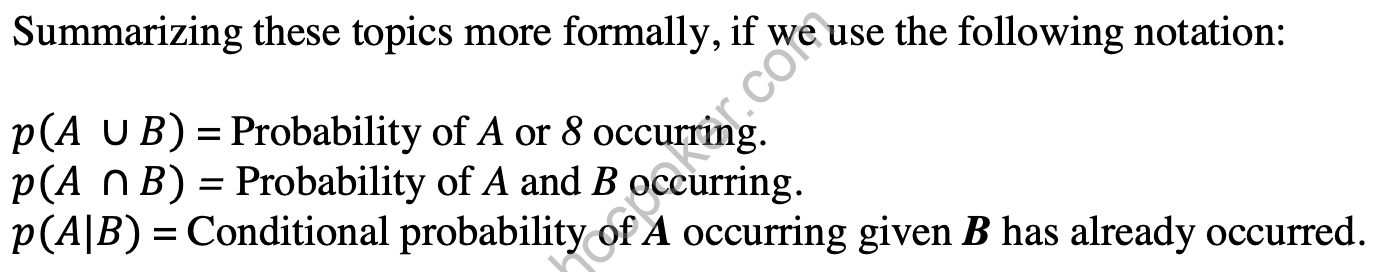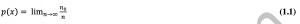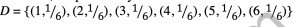# Dependent EventsSome events, by contrast, do have impacts on each other. For example, before a baseball game, a certain talented pitcher might have a 3% chance of pitching nine innings and allowing no runs, while his team might have a 60% chance of winning the game. However, the chance of the pitcher’s team winning the game and him also pitching a shutout is obviously not 60% times 3%. Instead, it is very close to 3% itself, because the pitcher’s team will virtually always win the game when he accomplishes this. These events are called dependent. We can also consider the conditional probability of A given B, which is the chance that if B happens, A will also happen. The probability of A and B both occurring for dependent events is equal to the probability of A multiplied by the conditional probability of B given A. Events are independent if the conditional probability of A given B is equal to the probability of A alone.

The ∪ and ∩ notations are from set theory and formally represent “union” and “intersection.” We prefer the more mundane terms “or” and “and.” Likewise, | is the symbol for “given,” so we pronounce these expressions as follows:

We can now return to the question at hand. How frequently will a single holdem hand dealt from a full deck contain two aces? There are two events here:

• A: The first card is an ace.
• B: The second card is an ace.

However, these two events are dependent, if A occurs (the first card is an ace), then it is less likely that B will occur, as the cards are dealt without replacement. So p(B|A) is the chance that the second card is an ace given that the first card is an ace. There are three aces remaining, and fifty-one possible cards, so

There are a number of other simple properties that we can mention about probabilities. First, the probability of any event is at least zero and no greater than one. Referring back to the definition of probability, n trials will never result in more than n occurrences of the event, and never less than zero occurrences. The probability of an event that is certain to occur is one. The probability of an event that never occurs is zero. Tire probability of an event’s complement -that is, the chance that an event does not occur, is simply one minus the event’s probability.

Summarizing, if we use the following notation:

We can solve many probability problems using these rules
Some common questions of probability are simple, such as the chance of rolling double sixes on two dice, hi terms of probability, this can be stated using equation 1.3, since the die rolls are independent. Let p(A) be the probability of rolling a six on the first die and p(B) be the probability of rolling a six on the second die. Then:

Likewise, using equation 1.2, the chance of a single player holding aces, kings, or queens becomes:

Additionally we can solve more complex questions, such as:

How likely is it that a suited hand will flop a flush?

We hold two of the flush suit, leaving eleven in the deck. All three of the cards must be of the flush suit, meaning that we have A the first card being a flush card, B the second card being a flush card given that the first card is a flush card, and C= the third card being a flush card given than both of the first two are flush cards.

Applying equation 1.5, we get:

We can apply these rules to -virtually any situation, and throughout the text we will use these properties and rules to calculate probabilities for single events.Previous post Decisions Under Risk: Probability and ExpectationNext post Probability Distributions